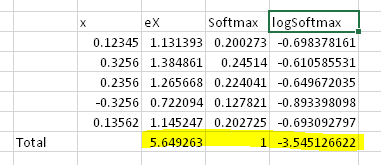LogSoftmax vs Softmax!

(Santhanam Elumalai) #1

What is an advantage or where it is appropriate to use the logsoftmax instead of softmax, I know logsoftmax is log(softmax) but softmax is meant to define the portion of the individual value in a group. But result of logsoftmax is not the same(ie the total of it is not 1 like the softmax). So where it exactly adds value.0 Likes

(Alex Lee) #2
``````def log_softmax(input, dim=None, _stacklevel=3):
r"""Applies a softmax followed by a logarithm.
While mathematically equivalent to log(softmax(x)), doing these two
operations separately is slower, and numerically unstable. This function
uses an alternative formulation to compute the output and gradient correctly.
See :class:`~torch.nn.LogSoftmax` for more details.
Arguments:
input (Variable): input
dim (int): A dimension along which log_softmax will be computed.
"""
``````